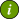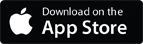### OSH Answers Fact SheetsEasy-to-read, question-and-answer fact sheets covering a wide range of workplace health and safety topics, from hazards to diseases to ergonomics to workplace promotion. MORE ABOUT >Search all fact sheets:

Type a word, a phrase, or ask a question

# Calculating Recommended Weight Limit (RWL)

## What is the Revised NIOSH lifting equation?

The equation is:

LC x HM x VM x DM x AM x FM x CM = RWL

where LC is the load constant (23 kg) and other factors in the equation are:

• HM, the Horizontal Multiplier factor
• VM, the Vertical Multiplier factor
• DM, the Distance Multiplier factor
• FM, the Frequency Multiplier factor
• AM, the Asymmetric Multiplier factor
• CM, the Coupling Multiplier factor
• RWL, the Recommended Weight Limit

For each value, look up the corresponding factor and use this number in the equation. See Assessing Relevant Handling Factors for explanation of terms.

To use the calculator, click on the values for each factor in the left hand column (or you can input your numbers directly into the second equation). Press the "Calculate RWL" button when you have finished selecting all of the values.

Horizontal Multiplier (HM): Horizontal distance (H, in cm) from the midpoint between the ankles to the hands while holding the object.

H = Horizontal Distance (cm) HM Factor
25 or less 1.00
300.83
40 0.63
50 0.50
60 0.42

Vertical Multipler (VM): The vertical distance (V, in cm) of the hands from the ground at the start of the lift.

V = Starting Height (cm) VM Factor
0 0.78
30   0.87
50  0.93
70   0.99
100   0.93
150   0.78
175  0.70
>175  0.00

Distance Multipler (DM): The vertical distance (D, in cm) that the load travels.

D = Lifting Distance (cm) DM Factor
25 or less 1.00
40   0.93
55   0.90
100   0.87
145  0.85
175  0.85
>175  0.00

Asymmetric Multipler (AM): The twisting angle (A) of the body while lifting, measured in degrees.

A = Angle (degrees) AM Factor
90°  0.71
60°   0.81
45°   0.86
30°  0.90
0°  1.00

Frequency Multipler (FM): The frequency (F) of lifts and the duration of lifting (in minutes or seconds) over a workshift.

F = Time Between Lifts  FM Factor
Lifting While Standing: OR Lifting While Stooping:
One Hour or Less Over One Hour One Hour or Less Over One Hour
5 min 1.00 0.85 1.00 0.85
1 min  0.94 0.75 0.94 0.75
30 sec 0.91 0.65 0.91 0.65
15 sec 0.84 0.45 0.84 0.45
10 sec0.750.270.750.27
6 sec0.450.130.45-
5 sec0.37 -0.37 -

FM = pick a value from (A) and (B).

(A) Time Between Lifts
Lifting While Standing

Lifting While Stooping

Coupling Multipler (CM): The quality of grasp (or coupling, C) classified as good, fair or poor and depends on the body position (either standing or stooping).

C = Grasp CM Factor:
Standing Stooping
Good (handles) 1.00 1.00
Fair 1.00 0.95
Poor 0.90 0.90

CM = pick a value from (A) and (B).

(A)

The revised NIOSH Lifting Equation is: 23 Kg x HM x VM x DM x AM x FM x CM= RWL
23 Kg x x x x x x =

or, input values directly in the equation below:
23 Kg x x x x x x =